1 / 10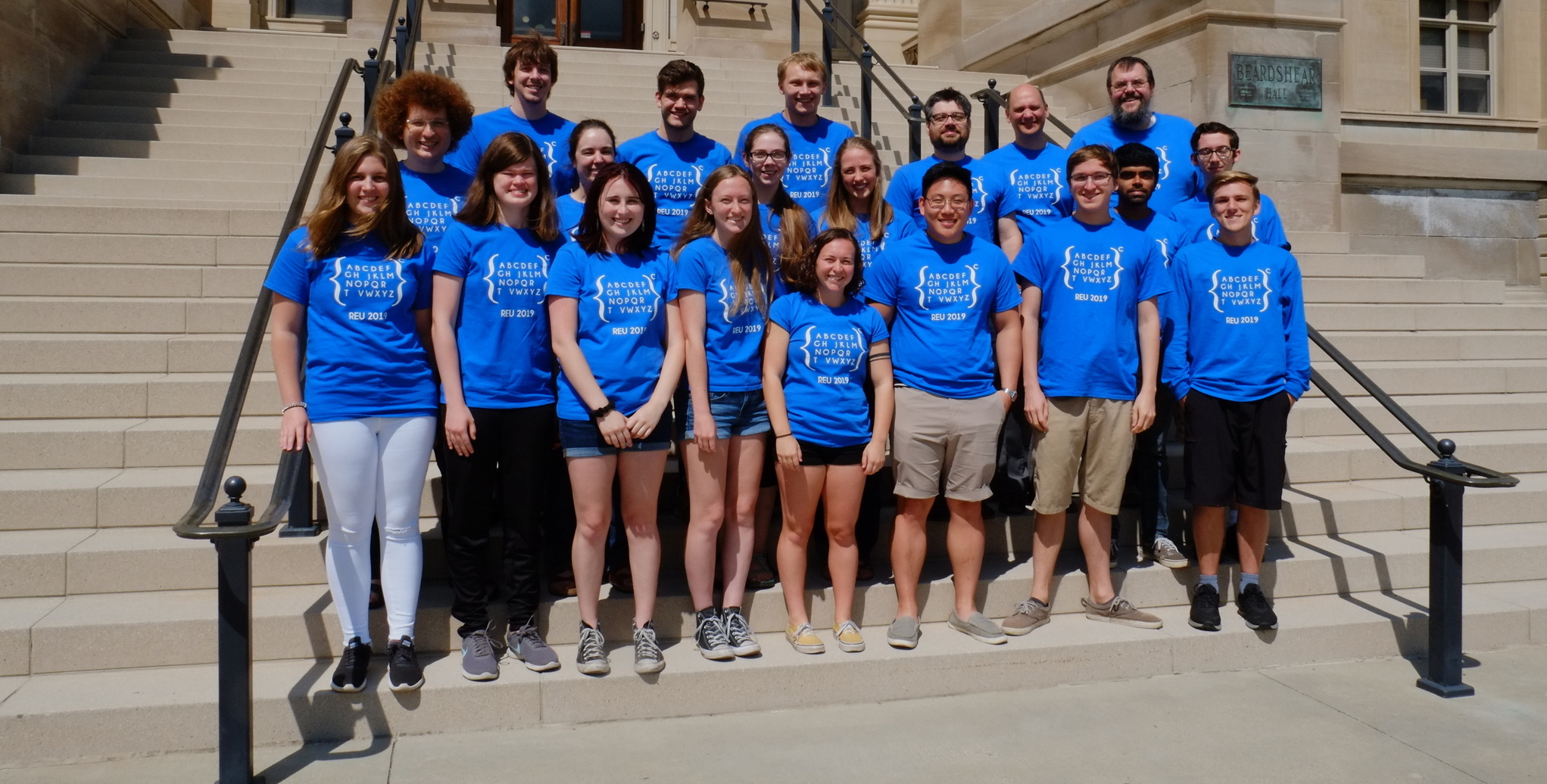Math REU @ ISU - Summer 2019
2 / 10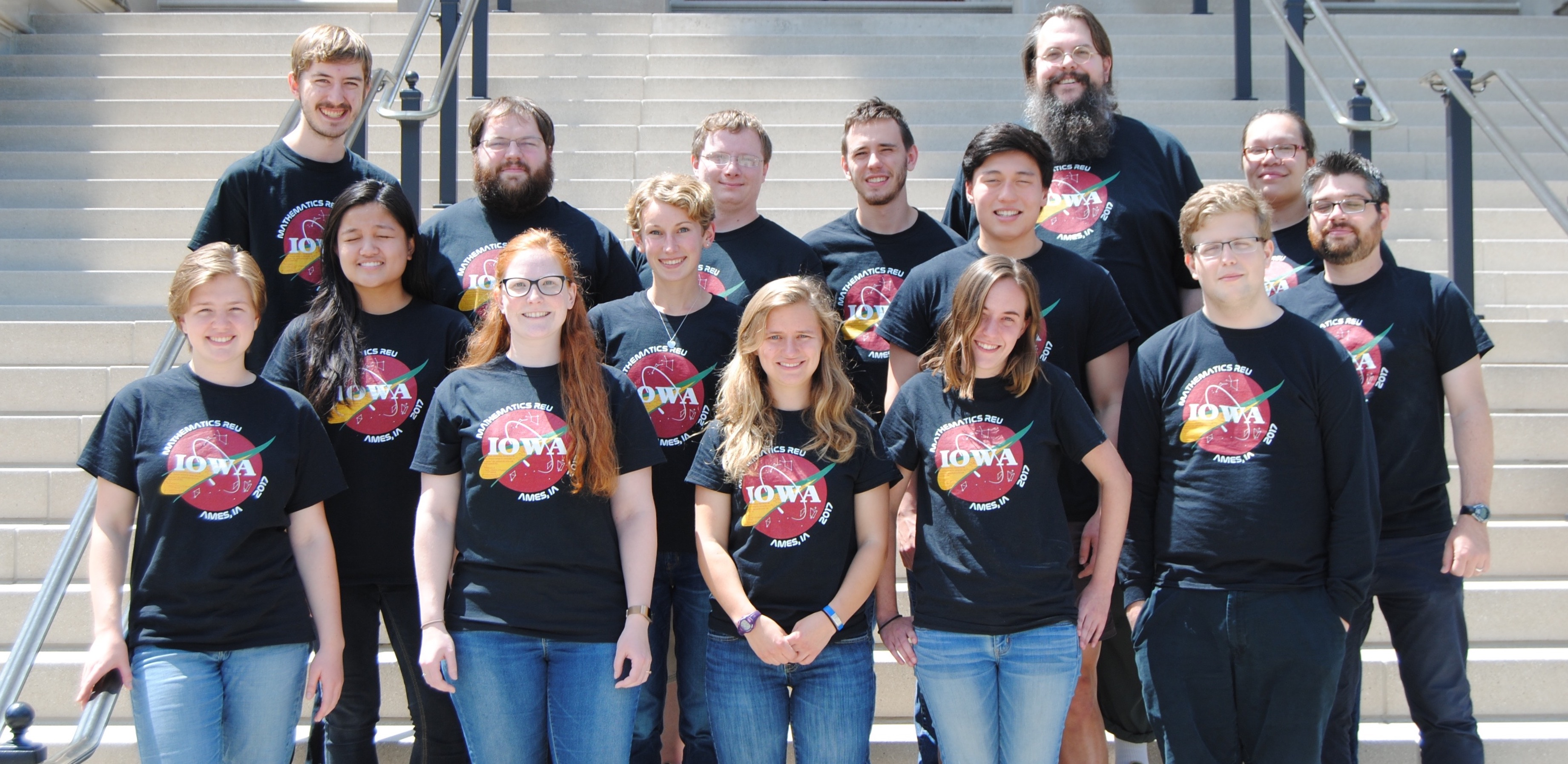Math REU @ ISU - Summer 2017
3 / 10Math REU @ ISU - Summer 2015
4 / 10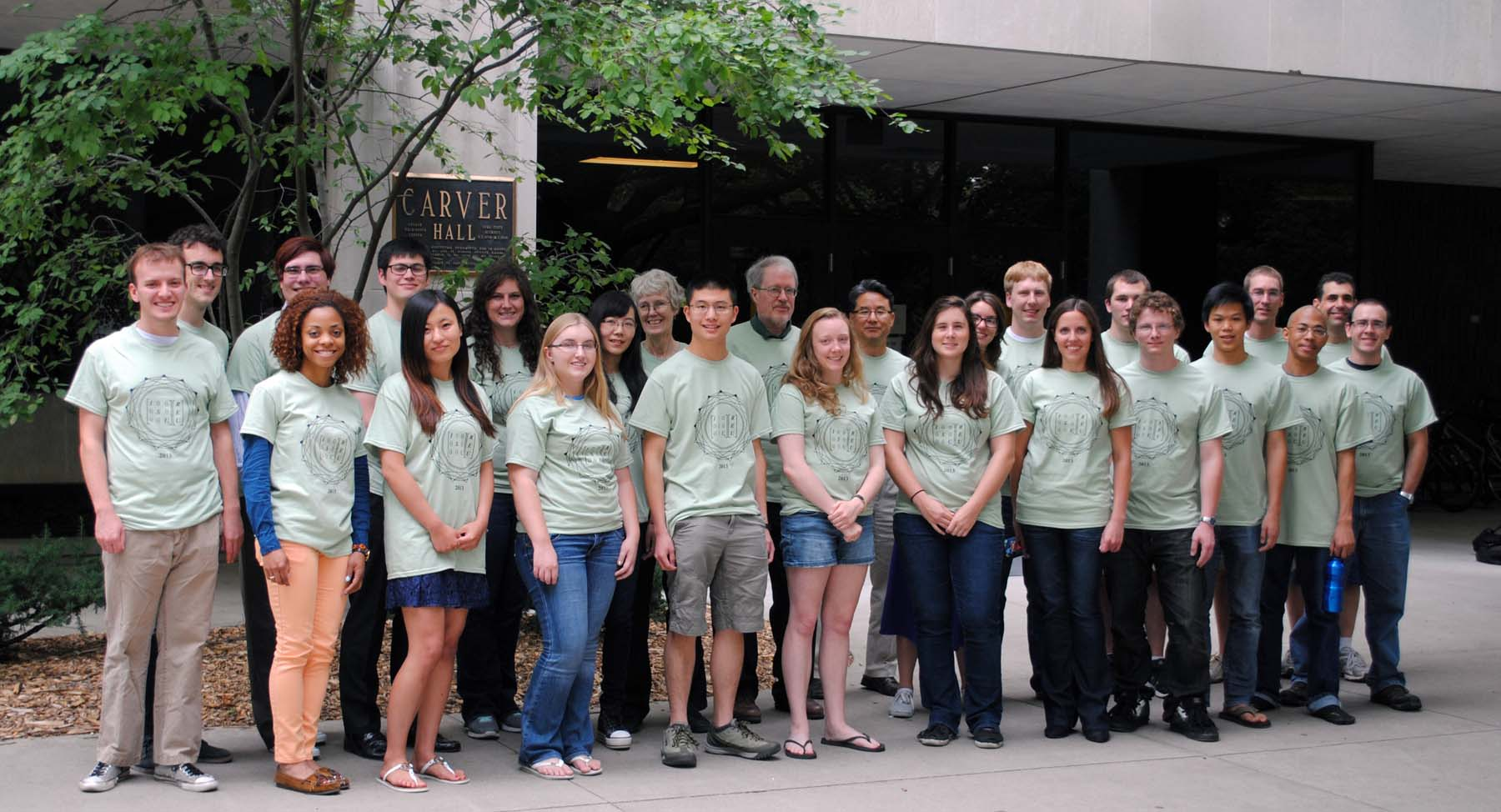Math REU @ ISU - Summer 2013
5 / 10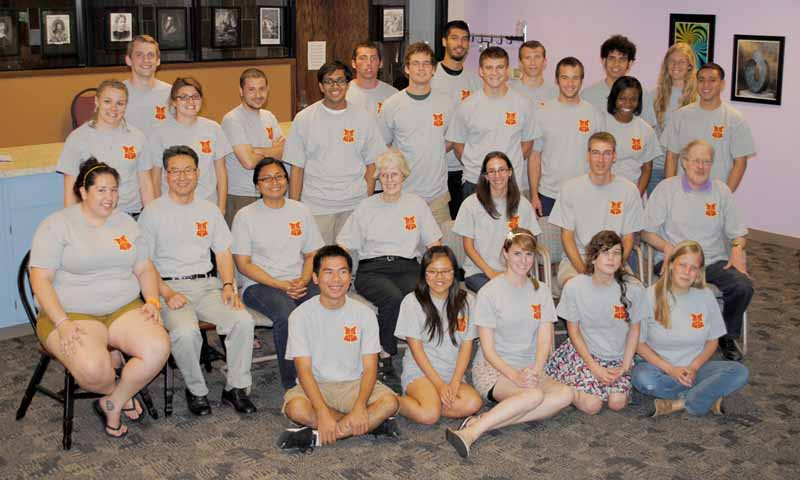Math REU @ ISU - Summer 2011
6 / 10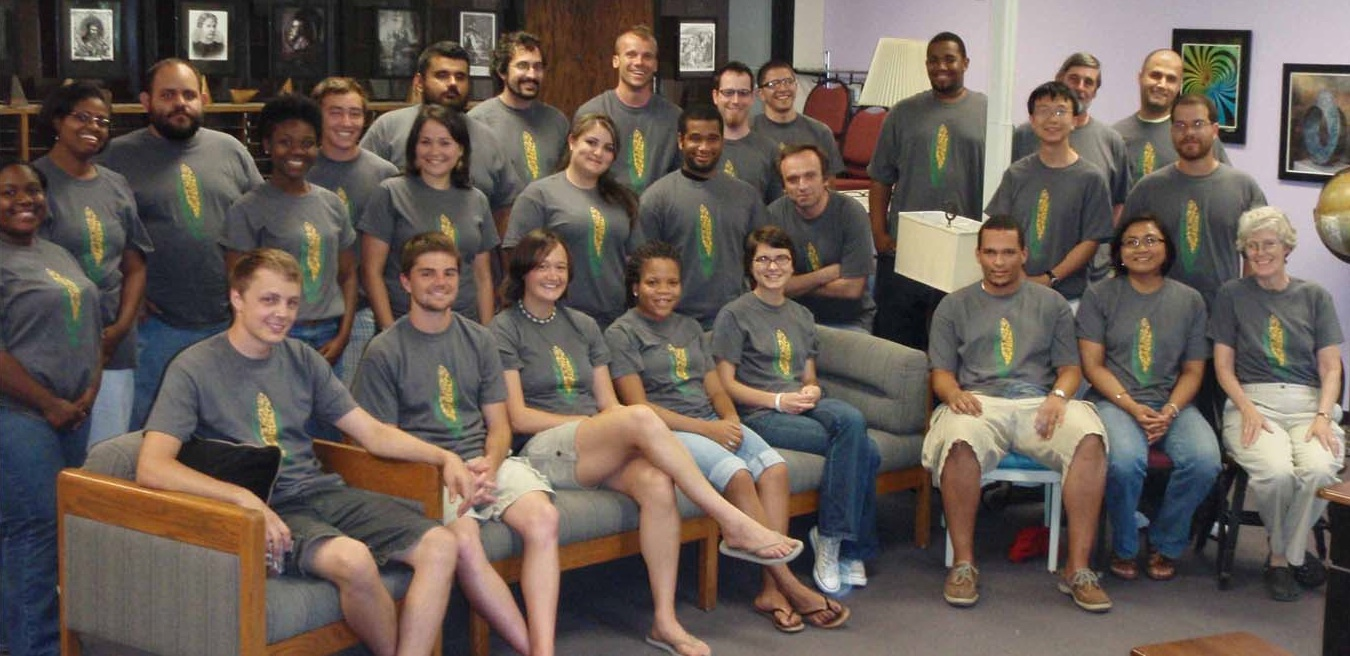Math REU @ ISU - Summer 2010
7 / 10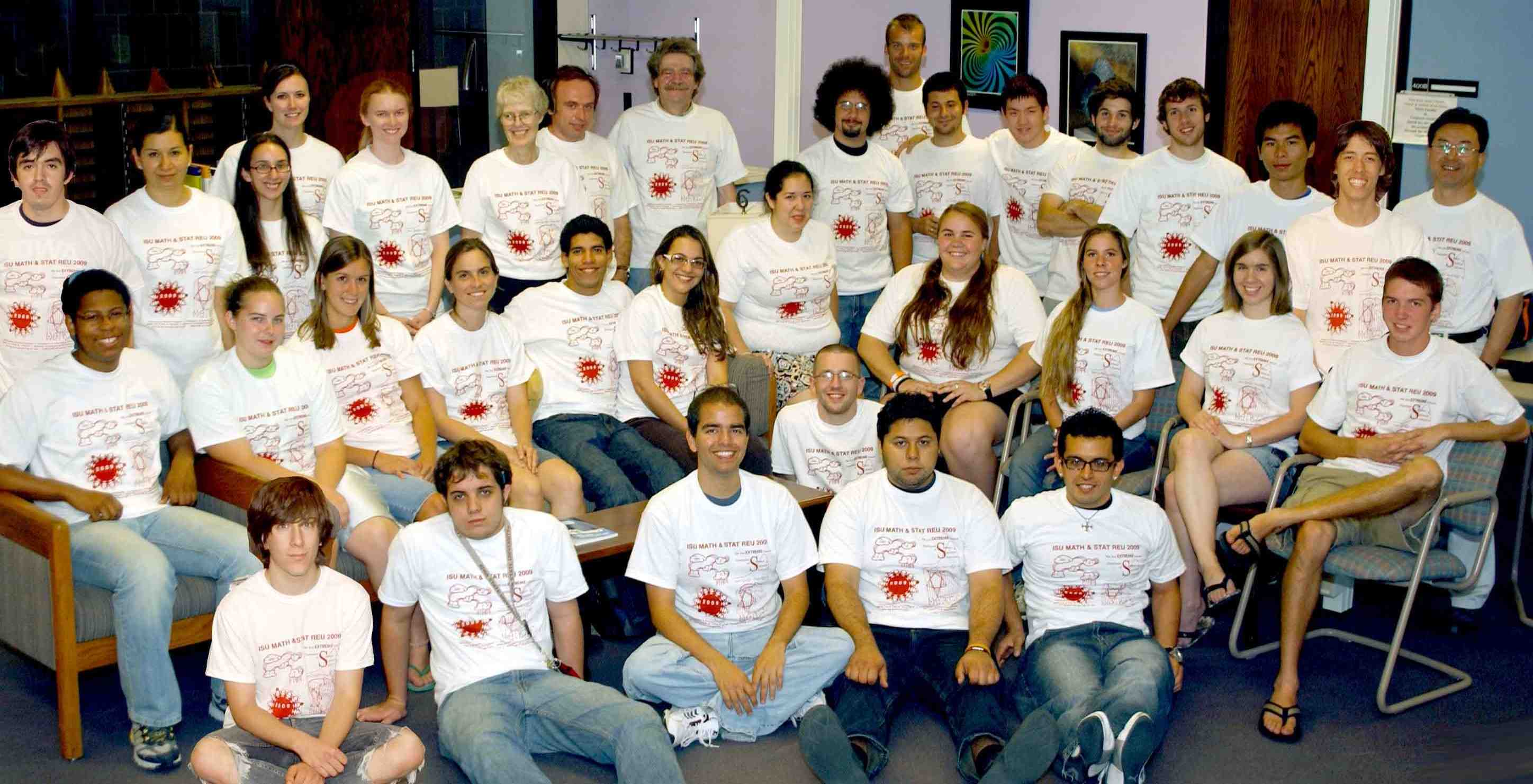Math REU @ ISU - Summer 2009
8 / 10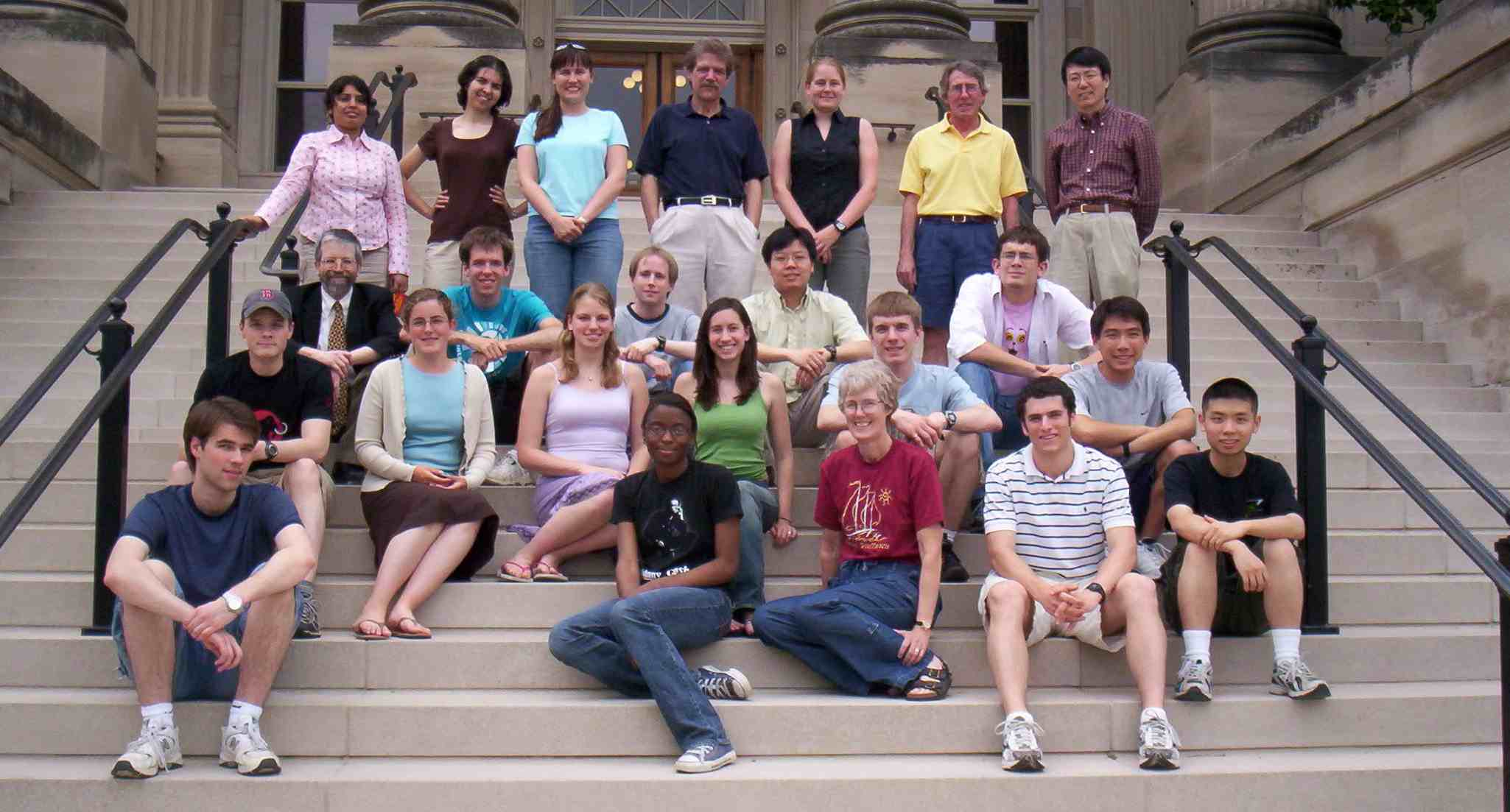Math REU @ ISU - Summer 2006
9 / 10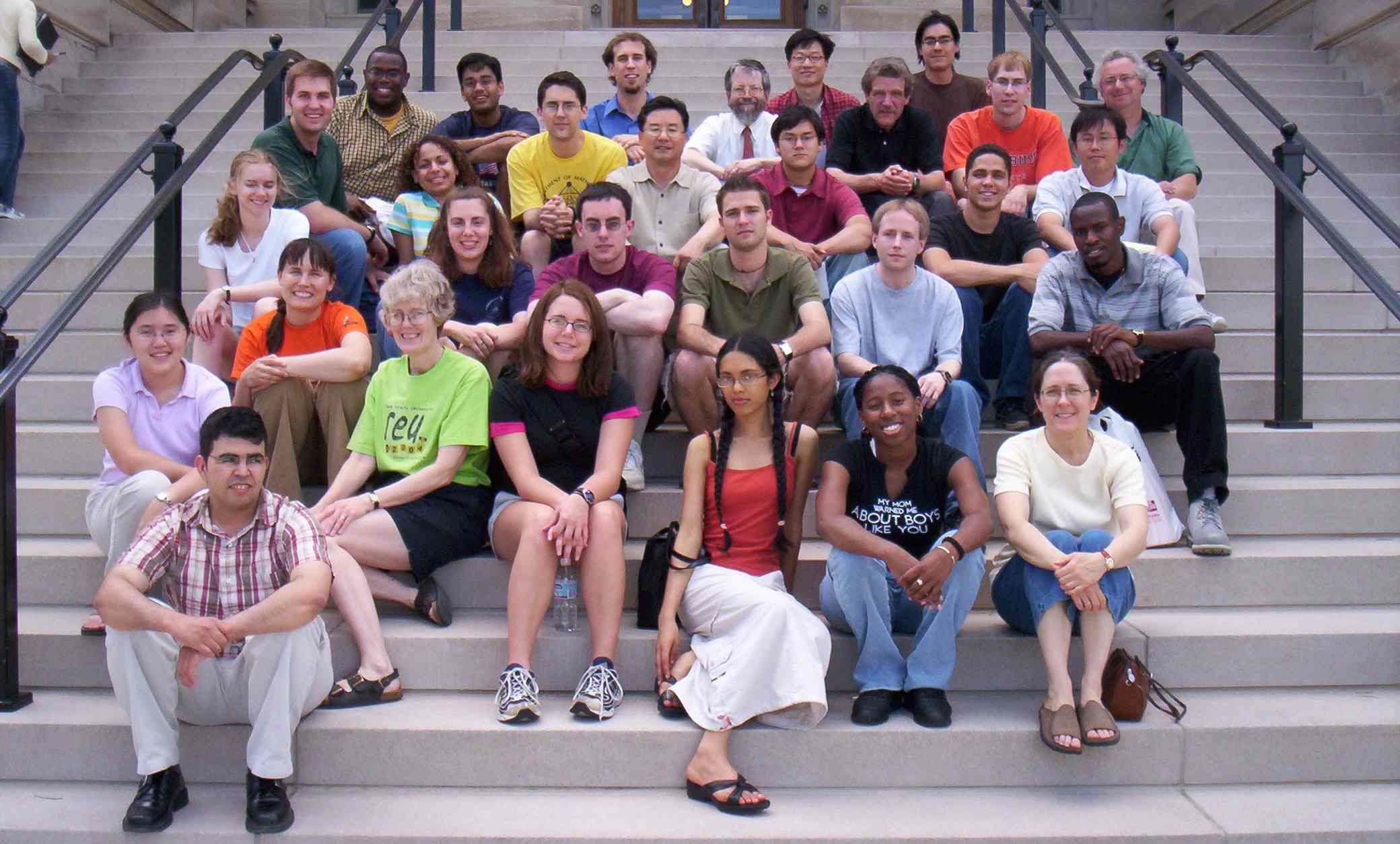Math REU @ ISU - Summer 2005
10 / 10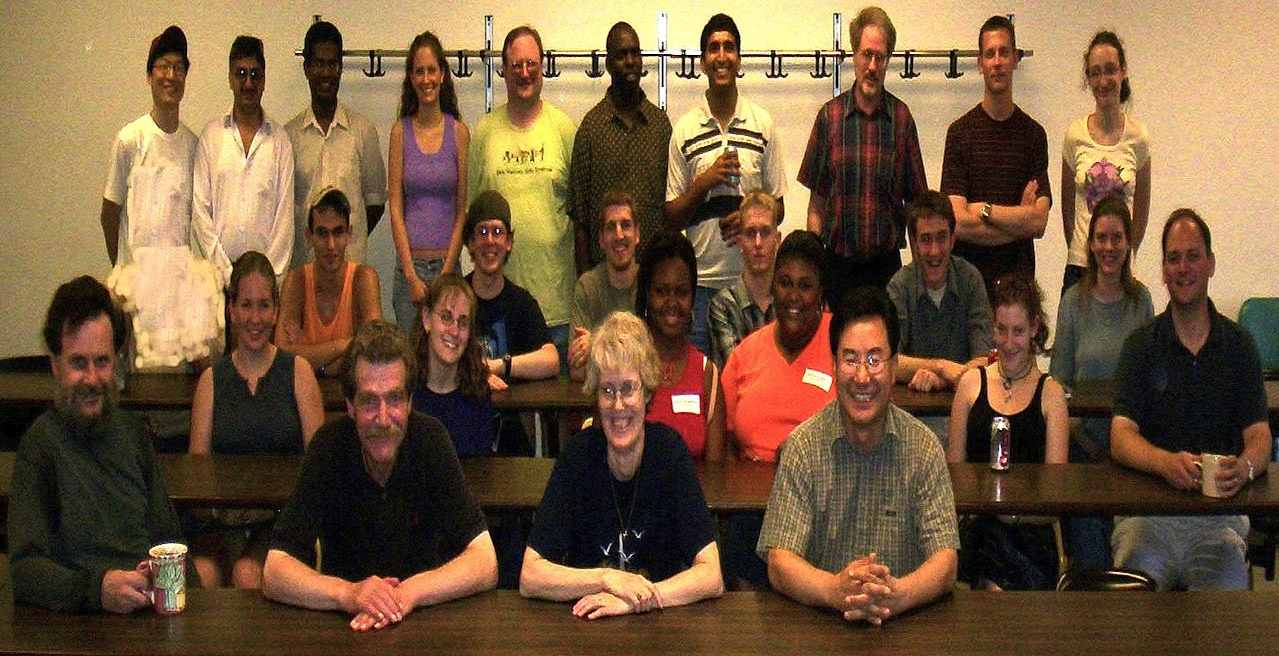Math REU @ ISU - Summer 2004

# 2022 Projects

## Temporal Logic Group:

Project Title: Temporal logic

Project Description:

A temporal logic is a formal system of rules and symbolism that allows us to represent, and reason about, propositions qualified in terms of time. In computer science, one of the most popular and widely used temporal logics is the linear time temporal logic (LTL), for which time is conveiced as a linear discrete succession of time instants. LTL language extends the classical propositional language by adding temporal operators $$G$$, $$X$$, and $$U$$, with intended meaning as follows:

• $$Gp$$: "It will always be the case that $$p$$"
• $$Xp$$: "Next time will be the case that $$p$$"
• $$pUq$$: "$$p$$ will be the case until a time when $$q$$ is the case"

This logic is used to express properties that describe specifications of a system (aka descriptions of the behaviors the system should have), such as safety, liveness, and fairness of certain behaviors. For example, the liveness specification “Every request is followed by a grant” can be formalized in LTL as $$G(\text{request} \rightarrow \neg G \neg \text{grant})$$.

LTL formulas are interpreted over the set of natural numbers $$\mathbb{N}$$ with its usual order $$\leq$$, where each number represents a time instant and zero represents the current one, plus an interpretation function (aka a computation) $$V$$ that assigns to each proposition $$p$$ a set of time instants $$V(p) \subseteq \mathbb{N}$$ on which it holds. A natural question over an LTL formula is to determine wether or not it is satisfiable (i.e. that is true in some interpretation), as well as determining if a given formula is a tautology (i.e. is always true regardless of its interpretation). This project will investigate algorithmic methods to produce "truth tables" for LTL formulas, as well as explore visualizations for the interpretations for such formulas.

Prerequisites:

Basic programming skills, some background in discrete mathematics (introductory undergraduate level), and mathematical maturity. Familiarity with concepts from mathematical logic (satisfiability, soundness, completeness, compactness, etc.) is desirable.

## Networks of Interacting Particle Systems Group:

Project Title: Networks of Interacting Particle Systems

Project Description:

A network of interacting particle systems is given by a collection of stochastic processes that interact with their neighbors according to certain underlying network structure. In practice, such stochastic processes may denote membrane potentials of neurons, wealth of companies, status of individuals, properties of chemical molecules, etc.

This project will numerically investigate the effect of the network structure on the large population and long-time behavior of networks of interacting particle systems. A particular emphasis will be on random and heterogeneous networks. Such networks are usually analyzed through the adjacency matrix or graphon. Some models and questions to be considered include:

1. Discrete-time or continuous-time Markov chain, such as an SIR model of an epidemic: How would the network structure, representing the interaction between individuals, affect the spread of virus or flatten the curve?
2. Diffusion processes driven by Brownian motions: How would the network structure affect the stationary distribution of the system? How to efficiently simulate the system, through e.g. Euler or Euler-Maruyama discretization?

Prerequisites:

Probability and basic programming skills. Some background in graph theory, MATLAB, stochastic processes will be helpful but not required.

## Modifying Kemeny's Constant Group:

Project Title: Modifying Kemeny's Constant

Project Description:

A graph is a mathematical object that consists of vertices (or nodes) and the connections between them. Graphs are great tools for modeling different types of network systems like subway systems where the nodes are stations and the connections are tracks between them or the internet where the nodes are webpages and the connections are links between them. For many networks, we want to measure how connected they are which is generally a difficult task. One way to measure connectedness is to imagine a random walker and measure how long it takes them to get to different nodes. In the example of the subway system, we can think of a lost tourist who is randomly riding the subway trains trying to get to a particular station and the length of their trip is the number of trains that they take.

We can find the weighted average of length of a random walk from one node as a way to measure the connectedness of the entire network system. Surprisingly, this does not depend on the starting node and is called Kemeny’s constant. This measure of connectedness can also be computed using linear algebra tools. The probability transition matrix is a matrix where the value is the probability that our lost tourist will travel from one station to another by taking one train (hence probability is zero between stations that are not directly connected) and Kemeny’s constant is found using the spectral properties of this matrix.

In this project, we will compute Kemeny’s constant for a network and explore techniques to manipulate the network to achieve a desired Kemeny’s constant.

Prerequisites:

• Linear algebra
• Some experience in Python or SAGE is preferred

## Hearing the Shape of a Tree Group:

Project Title: Hearing the Shape of a Tree

Project Description:

A classic problem in mathematics is whether one can "hear the shape of a drum". The basic idea is whether some indirect measurements are sufficient to capture structure. For graphs (which looks at the connections (edges) between objects (vertices)) this question has focused on whether the spectrum (=set of eigenvalues) of a matrix associated with a graph is unique to that graph, or if there are other distinct graphs which have the same spectrum.

Schwenk showed that almost all trees have a distinct (=nonisomorphic) tree for which the two trees have the same eigenvalues for the adjacency matrix ($$A$$). A small modification shows that almost all trees have a distinct non-tree for which the two graphs have the same eigenvalues. We will call a graph which is not a tree but has the same spectrum as a tree a "spectral-faux tree". So for the adjacency matrix almost all trees are cospectral with a spectral-faux tree. On the other hand for the Laplacian matrix ($$L=D-A$$ where $$D$$ is the diagonal matrix of degrees) it can be readily shown that there are no spectral-faux trees.

Our initial goal will be to consider other possible matrices and determine what can be said about the existence and/or prevalence of faux trees. This will require finding new cospectral constructions as well as proofs for why certain objects cannot exist.

Prerequisites:

• Basic linear algebra with proofs
• Basic graph theory and/or combinatorics
• Experience with SAGE and/or Python is desirable